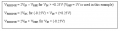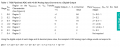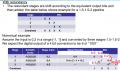# visualising redundancy in1.5bit pipe line ADC

#### yef smith

Joined Aug 2, 2020
397
0
In pipe line ADC we have redundancy where we increase number of levels to somehow more accuratly measure the Input and reduce the error. IN the manual shown bellow we see redundancy example from MAXIM manual , But they dont shown in the example how exactly given an error they redundancy fixes it? they only say that we fix problem by doing right shift to the bits and summing the stages data. I thing digital fixing is not redundancy i cant see how in a case of an error redundancy helps me with figuring the correct location of digital value. Example would be great! Thanks.#### Beau Schwabe

Joined Nov 7, 2019
130
A right shift is a divide by two ... so summing two stages before a right shift essentially just adds two numbers and divides by two to produce an average of those two numbers.

Averaging increases the Signal to Noise ratio by minimizing random noise, while re-enforcing a true signal or bias level.

The signal to noise ratio via "Ensemble Averaging" works out to the square root of the number of samples. i.e. If you sample 9 times, then the S/N ratio will increase by a factor of 3:1 .... 3 being the square root of 9

4 samples 2:1
2 samples 1.41:1 <-- note only a 41% increase in your signal to noise ratio

#### yef smith

Joined Aug 2, 2020
397
Hello, So given an example here 1.5bit->1.5bit->2bit
first stage gived us b5b4
seconds stage gives us b3b2
third stage gives us b1b0
What bits you consider to represent the same data?
Those bits came from different stages.next stage gets different "input"(residue)

Binary avarage is implemented as avg = (x & y) + ((x ^ y) >> 1);
I cant see it here in the table bellow.Last edited: